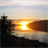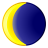# Possible to calculate mean over several fields?

459
1
03-27-2013 04:41 PMNew Contributor II
Hi all,

I have a data set which is based on a survey where hundreds of individuals were asked to list sources of income. The first column is respondent # and the next four columns are the various categories of income, which are populated with binary values (i.e. 0=not a source of income, 1=yes is a source of income). Is it possible to calculate what the mean is across the four field? I'm only familiar with calculating mean within a single column.

Thanks in advance,

David
Tags (2)
1 ReplyOccasional Contributor
I may not understand what you actually want to do... so forgive me if I'm off the track.  I'm understanding that you have 4 binary fields and want to know the average number of 1's (across those fields) for each respondent.  So if respondent A has 2 zeros and 2 ones you would like to know that the average is 0.5.  Is that correct?  If so, you can use Calculate Field... create your new Mean1s field, then calculate it as the sum of the 4 fields, divided by 4.
Hope this helps!
Lauren
Esri
Geoprocessing and Analysis